# The Spectrum of Atomic Hydrogen

NOTE

This manual describes the laboratory experiment used during the 1996 - 1997 academic year. Significant changes have been made since then, and the manual used during the current academic year is in NOT available yet on the WEB. Hardcopies can be purchased at the bookstore.

## Purpose

To verify the quantum nature for the Balmer series, specifically for hydrogen and sodium.

## Introduction

One of the earliest successes of quantum mechanics was the explanation of the spectrum of atomic hydrogen. It has long been known that atomic hydrogen had some regular features in its spectrum. These regularities can be expressed as,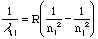, (1)

where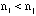are both integers and R is a constant. The spectral lines are classified into series which are sets of lines with a common value of the integer. Thus we have written the expression as the wave number (inverse wavelength usually quoted in units of cm-1) of the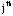member of the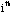series. The various series of the spectrum of atomic hydrogen are listed in Table 1.

According to the Bohr theory (and of more formal quantum mechanics), the Rydberg constant R is given to a first approximation by,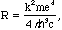(2)

where e and m are the electron charge and mass, respectfully, c is the velocity of light, is Planck's constant divided by 2[[pi]], and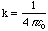. You may find an elementary discussion of the derivation of this expression in many texts (e.g. Eisberg's Fundamentals of Modern Physics, Chapter 5, sections 1, 2, and 3). There is a small correction which takes into account the mass M of the nucleus. This involves replacing m with a reduced mass u given by,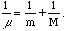(3)

```Name                  Wavelength Range      Series Expression
---------------------------------------------------------------------------
Lyman                 Ultraviolet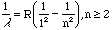Balmer                Near UV & Visible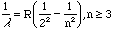Paschen               Infrared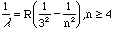Brackett              Infrared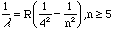Pfund                 Infrared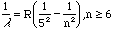---------------------------------------------------------------------------```

Table 1

Examine the spectrum of atomic hydrogen in the visible region. This will restrict your test of the theory to three or four lines of the Balmer Series, examine the chart on the wall of the laboratory which should be sufficient to verify the quantum nature of the expression for the Balmer series and allow you to obtain a value of the Rydberg Constant using the series expression given in Table 1.

## Prelab Homework

The prelab homework must be done at home and handed to TA before you start the lab. Read the lab instructions for this lab.

### Question

Write down the ranges of the wavelengths in nanometers(nm) for the regions of the spectrum usually designated by x-ray, ultraviolet, visible, infrared and microwave, radio.

## Part I: Alignment of Spectrometer

The spectrometer needs to be aligned to ensure decent measurements of the sodium, also on the chart on the wall, and hydrogen spectral lines. The spectrometer is shown in Figure 1. Below the telescope there is a pair of knobs. If you loosen the top knob, then the telescope will be free to move. Loosening the bottom knob allows the platform to rotate. If you rotate the platform, then the angles visible in the window on the platform will change. Keep careful track of these changes.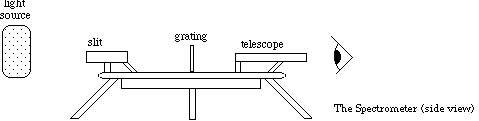Figure 1

### Procedure

• To align the spectrometer, first focus the telescope at infinity any object on the lab wall. See Figure 2.

• With the grating removed from the spectrometer, adjust the slit and collimator for a sharp, narrow image of the source (slit). DO NOT REFOCUS THE TELESCOPE.

• Center the crossbar on the slit image.

• Record the angle displayed in the window. Be sure to use the same window throughout the lab. The instructor will explain how to read the vernier scale (the bottom scale is given in degrees and the top is given in arc seconds).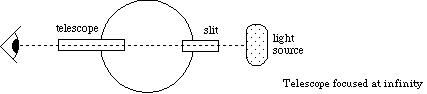Figure 2

• Insert the grating making sure that the grating lines are vertical to the platform. With the grating fixed in place, rotate the telescope 90deg. as shown in Figure 3. Fix the telescope in place.Figure 3

• Rotate the grating until the sodium light source (zero order spectrum--the light bulb and not the spectral lines) is centered on the crosshair. The incident angle of the light on the grating is 45deg..

• Fix the grating in place making sure that the crosshairs stay centered on the light.

• Turn the telescope 90deg. back to its original position. Rotate the platform 135deg., so that the light from the source is normal to the grating. The crosshair should be centered on the slit. Record the new zero angle.

## Part II: Calibration of Spectrometer

Light passing through the diffraction grating is diffracted into directions [[theta]] given by,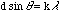, (4)

where d is the spacing between the lines on the grating, k is an integer, [[lambda]] is the wavelength of light, and [[theta]] is measured from the normal to the grating. (Figure 4).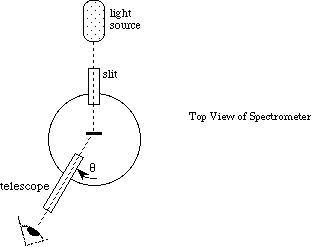Figure 4

### Procedure

• Determine the grating spacing d using the sodium D-lines. (The given line density on the grating is not necessarily accurate). The sodium D-lines are at 589.0 nm and 589.6 nm With care you should be able to resolve these two lines (note: they are yellow; ignore the green sodium line). If you cannot resolve the sodium D lines, use the average wavelength.

• Knowing the angle [[theta]] and the wavelength [[lambda]], you can determine d. Do this on both sides of your zero degree mark.

• Average your results for a final value of d.

## Part III: Balmer Series

### Procedure

• Replace the sodium source with the hydrogen source and measure the angles at which the hydrogen emission lines are seen.

• Record the positions of all observable lines, to both the left and the right of the zero position, for both first(k=1) and second(k=2) orders. (see Figure 5). Note that k=1 contains several different colored lines, k=2 contains the same number of colored lines.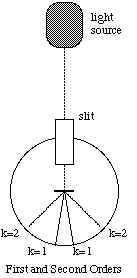Figure 5

## Data Analysis

From your data determine the wavelengths of the observed lines, and fit these to the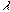obtained from the Balmer series expression for n=3,4 & 5.

### Question

Ordinary hydrogen has a nucleus consisting of one proton. Naturally occurring hydrogen, however, contains a small amount of deuterium, i.e., hydrogen having a nucleus consisting of a proton and a neutron. If the deuterium existed in sufficient quantity in your source to produce observable spectral lines, would you expect to resolve its lines from those of ordinary hydrogen with our equipment?

Send comments, questions and/or suggestions via email to wolfs@nsrl.rochester.edu.